Right angle and straight angle

Chapter 5 Class 6 Understanding Elementary Shapes
Concept wise

Suppose we have a map with North, South, East, West Map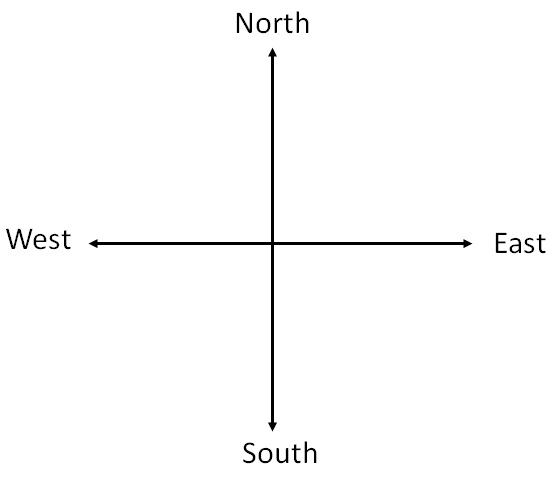If we move from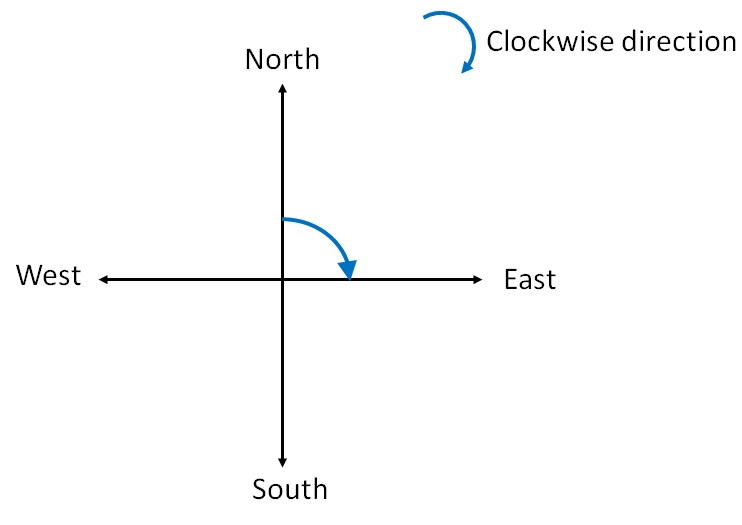We have rotated ‘right angle’ in clockwise direction.

We see that

We have rotated 1/4 of the full turn.

So, right angle is 1/4 of the full rotation

If we go from

East to South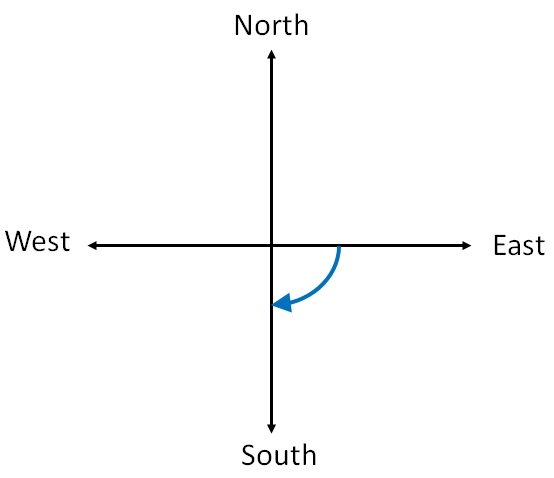We have rotated right angle in clockwise direction

Now, if we go from

South to North clockwise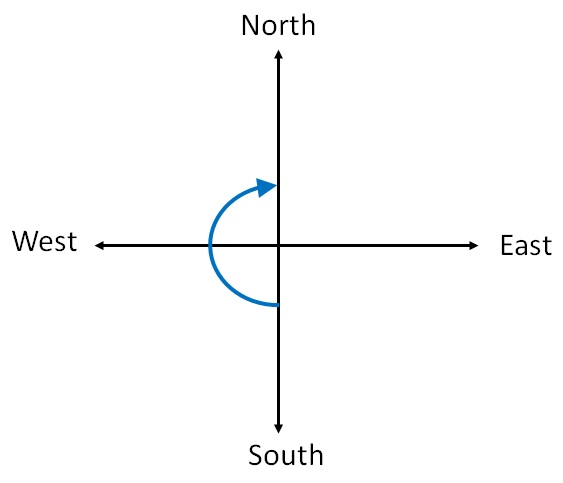We have rotated 1/2 revolutions.

This is straight angle .

2 right  angle = 1 straight angle

Similarly,

If we go from East to West Clockwise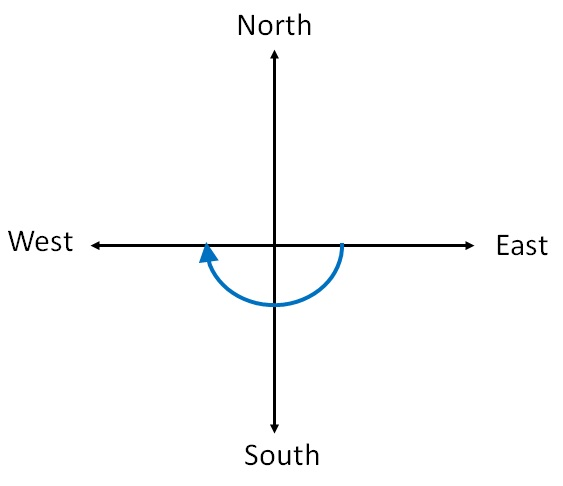Then we have rotated 1/2 revolution

i.e.  we have rotated

1 straight angle

Suppose,

We go from North to North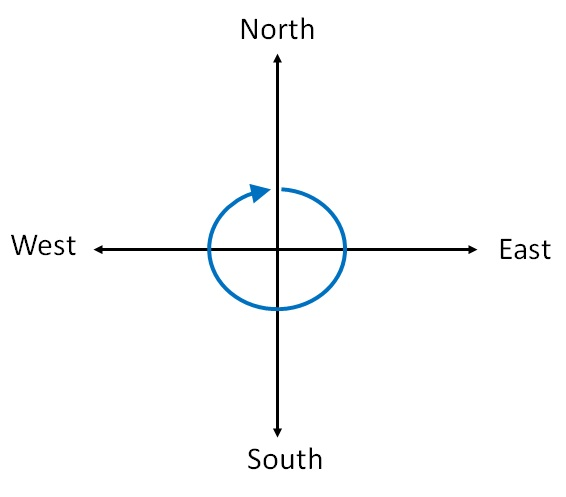In this case,

we have rotated 1 revolution

∴  we have rotated 1 full angle

#### In Summary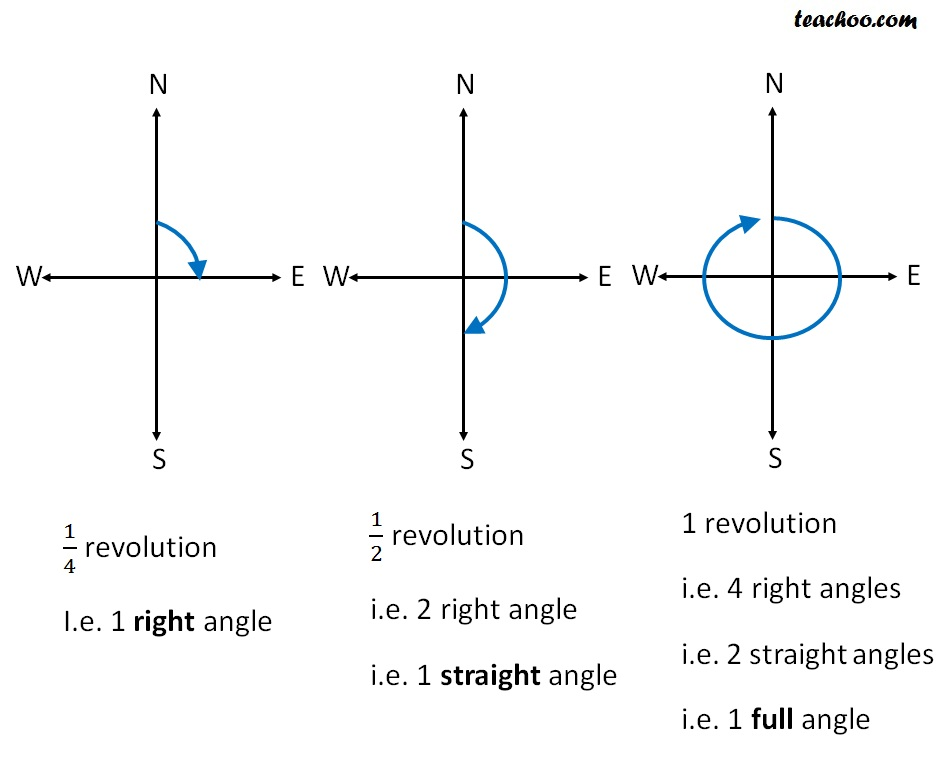Learn in your speed, with individual attention - Teachoo Maths 1-on-1 Class

### Transcript

In Summary, 1/4 revolution I.e. 1 right angle 1/2 revolution i.e. 2 right angle i.e. 1 straight angle 1 revolution i.e. 4 right angles i.e. 2 straight angles i.e. 1 full angle